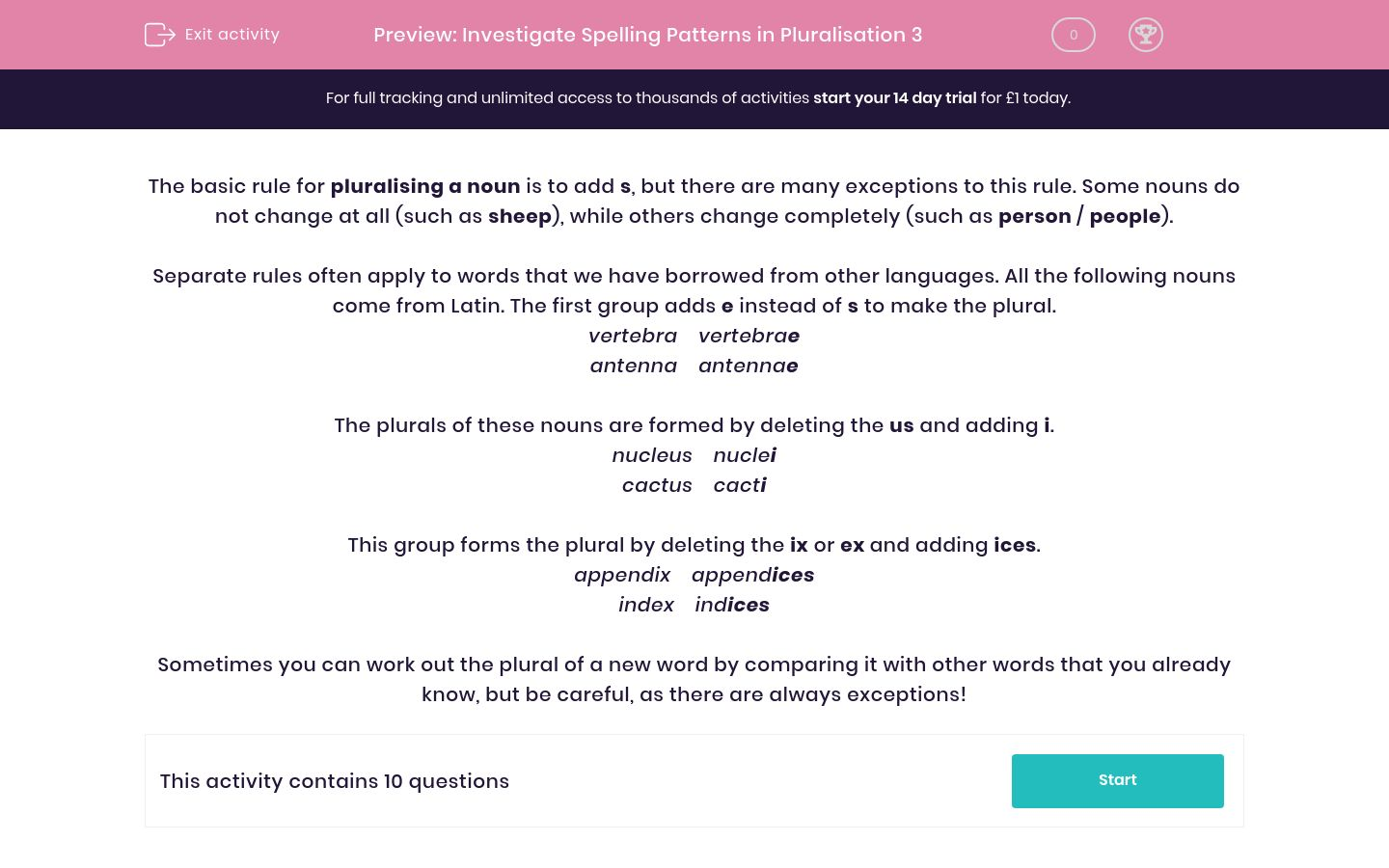# Investigate Spelling Patterns in Pluralisation 3

In this worksheet, students consider ways of pluralising nouns that have come from other languages.Key stage:  KS 2

Curriculum topic:   English

Curriculum subtopic:   Spelling: Plurals

Difficulty level:### QUESTION 1 of 10

The basic rule for pluralising a noun is to add s, but there are many exceptions to this rule. Some nouns do not change at all (such as sheep), while others change completely (such as person / people).

Separate rules often apply to words that we have borrowed from other languages. All the following nouns come from Latin. The first group adds e instead of s to make the plural.

vertebra    vertebrae

antenna    antennae

The plurals of these nouns are formed by deleting the us and adding i.

nucleus    nuclei

cactus    cacti

This group forms the plural by deleting the ix or ex and adding ices.

appendix    appendices

index    indices

Sometimes you can work out the plural of a new word by comparing it with other words that you already know, but be careful, as there are always exceptions!

Write down the plural of the following noun. You can work it out by comparing it with the nouns in the introduction page.

fungus

Write down the plural of the following noun. You can work it out by comparing it with the nouns in the introduction page.

formula

Write down the plural of the following noun. You can work it out by comparing it with the nouns in the introduction page.

matrix

Write down the plural of the following noun. You can work it out by comparing it with the nouns in the introduction page.

larva

For the next six questions you need to decide whether the noun follows the rules given in the introduction or is an exception.

What is the plural of crucifix?

Decide whether the noun follows the rules given in the introduction or is an exception.

What is the plural of octopus?

Decide whether the noun follows the rules given in the introduction or is an exception.

What is the plural of genius?

Decide whether the noun follows the rules given in the introduction or is an exception.

What is the plural of bonus?

Decide whether the noun follows the rules given in the introduction or is an exception.

What is the plural of suffix?

Decide whether the noun follows the rules given in the introduction or is an exception.

What is the plural of focus?

• Question 1

Write down the plural of the following noun. You can work it out by comparing it with the nouns in the introduction page.

fungus

fungi
• Question 2

Write down the plural of the following noun. You can work it out by comparing it with the nouns in the introduction page.

formula

formulae
• Question 3

Write down the plural of the following noun. You can work it out by comparing it with the nouns in the introduction page.

matrix

matrices
• Question 4

Write down the plural of the following noun. You can work it out by comparing it with the nouns in the introduction page.

larva

larvae
• Question 5

For the next six questions you need to decide whether the noun follows the rules given in the introduction or is an exception.

What is the plural of crucifix?

crucifixes
• Question 6

Decide whether the noun follows the rules given in the introduction or is an exception.

What is the plural of octopus?

octopuses
octopi
EDDIE SAYS
Both \'octopuses\' and \'octopi\' are used, but the correct plural is really \'octopuses\' as the word comes from Greek and not Latin.
• Question 7

Decide whether the noun follows the rules given in the introduction or is an exception.

What is the plural of genius?

geniuses
genii
EDDIE SAYS
Both \'geniuses\' and \'genii\' are correct! Most people use the word \'geniuses\' these days.
• Question 8

Decide whether the noun follows the rules given in the introduction or is an exception.

What is the plural of bonus?

bonuses
• Question 9

Decide whether the noun follows the rules given in the introduction or is an exception.

What is the plural of suffix?

suffixes
• Question 10

Decide whether the noun follows the rules given in the introduction or is an exception.

What is the plural of focus?

foci
focuses
EDDIE SAYS
Both \'focuses\' and \'foci\' are correct! As the word comes from Latin the plural can be \'foci\', but these days people tend to use the word \'focuses\' more often.
---- OR ----

Sign up for a £1 trial so you can track and measure your child's progress on this activity.

### What is EdPlace?

We're your National Curriculum aligned online education content provider helping each child succeed in English, maths and science from year 1 to GCSE. With an EdPlace account you’ll be able to track and measure progress, helping each child achieve their best. We build confidence and attainment by personalising each child’s learning at a level that suits them.

Get started